# AP Chemistry : Chemical Reactions

## Example Questions

← Previous 1

### Example Question #1 : Chemical Reactions

What is the net ionic equation for the reaction that occurs when sodium chloride is mixed with silver nitrate.

NaCl (aq) + AgNO3 (aq) -> NaNO3 (aq) + AgCl (aq)

NaCl (aq) + AgNO3 (aq)  ->  NaNO3 (s) + AgCl (aq)

Na+ (aq) + NO3- (aq) -> NaNO3 (s)

Ag+ (aq) + Cl- (aq) -> AgCl (s)

NaCl (aq) + AgNO3 (aq)  ->  NaNO3 (aq) + AgCl (s)

Ag+ (aq) + Cl- (aq) -> AgCl (s)

Explanation:

Only the Ag and Cl ions are reacting to form solid silver chloride.

### Example Question #2 : Chemical Reactions

What is the net ionic equation that occurs when sodium chloride is mixed with potassium nitrate?

NaCl (aq) + KNO3 (aq)  ->  NaNO3 (s) + KCl (aq)

Na+ (aq) + NO3- (aq) -> NaNO3 (s)

NaCl (aq) + KNO3 (aq)  ->  KNO3 (aq) + KCl (s)

NaCl (aq) + KNO3 (aq) -> NaNO3 (aq) + KCl (aq)

No Reaction

No Reaction

Explanation:

Since no precipitation is predicted by the solubility rules, there will be no reaction.

### Example Question #3 : Chemical Reactions

What is the net ionic reaction when potassium carbonate is mixed with Calcium bromide?

2 K+ (aq) + Br- (aq) -> 2 KBr (s)

2 K2CO3 (aq) + CaBr2 (aq) ->  2 KBr (aq)  +  CaCO3 (s)

Ca2+ (aq) + CO32- (aq) ->  CaCO3(s)

2 K2CO3 (aq) + CaBr2 (aq) ->  2 KBr (s)  +  CaCO3 (aq)

2 K2CO3 (aq) + CaBr2 (aq) ->  2 KBr (aq)  +  CaCO3 (aq)

Ca2+ (aq) + CO32- (aq) ->  CaCO3(s)

Explanation:

Only the calcium and carbonate ions are reacting according to the solubility rules to form calcium carbonate.

### Example Question #4 : Chemical Reactions

What is the net ionic reaction when a solution of Cd(NO3)2 is reacted with (NH4)2S?

Cd(NO3)2 (aq) + (NH4)2S (aq) ->  CdS (aq) + 2 NH4NO3 (aq)

Cd2+(aq) + S2- ->  CdS (s)

Cd(NO3)2 (aq) + (NH4)2S (aq) ->  CdS (s) + 2 NH4NO3 (aq)

NH4+ (aq) + NO3- (aq)  ->  NH4NO3 (s)

Cd(NO3)2 (aq) + (NH4)2S (aq) ->  CdS (s) + 2 NH4NO3 (aq)

Cd2+(aq) + S2- ->  CdS (s)

Explanation:

Only Cd and S ions are interacting according to the solubility rules to form a solid precipitant.

### Example Question #5 : Chemical Reactions

What is the net ionic reaction when a solution of rubidium sulfate is mixed with barium hydroxide?

Ba2+ (aq) + SO42- (aq)  ->  BaSO4 (s)

Rb2SO4 (aq) + Ba(OH)2 (aq)  ->  2 RbOH  (aq)  +  BaSO4 (s)

Rb+ (aq) + OH- (aq)  ->  RbOH (s)

No Reaction

Rb2SO4 (aq) + Ba(OH)2 (aq)  ->  2 RbOH  (s)  +  BaSO4 (aq)

Ba2+ (aq) + SO42- (aq)  ->  BaSO4 (s)

Explanation:

Only the Ba and sulfate ions will react according to the solubility rules.

### Example Question #6 : Chemical Reactions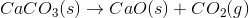The reaction above could be classified as __________.

decomposition

decomposition and oxidation-reduction

single displacement

synthesis

oxidation-reduction

decomposition

Explanation:

When one molecule is converted into two or more smaller molecules, the reaction is considered a decomposition reaction. In this example, none of the elements under go a change in oxidation number, so this is not an oxidation-reduction reaction. (Calcium is always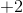, oxygen is always, and carbon remains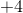throughout the reaction.)

### Example Question #7 : Chemical Reactions

Which of the following represents a combustion reaction?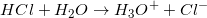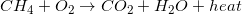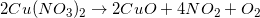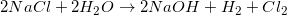Explanation:

Combustion reactions always produce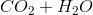and excess heat. Depending on the molecule that is oxidized by oxygen, which in this case was methane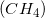, the ratios between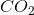and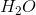can vary.

### Example Question #8 : Chemical Reactions

What is the chemical formula of the salt formed when a chemist mixes solvated Potassium and Arsenic ions in solution?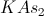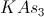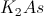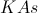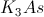Explanation:

Potassium is a Group I element, so to get to a filled valence shell, it will lost one electron, yielding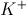.

Arsenic is a Group 5 element, so it needs to gain three electrons to obtain a filled valence shell, yielding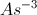.

In order to balance out the charges, the resultant salt will be.

### Example Question #9 : Chemical Reactions

What is the net ionic equation for the ion exchange reaction between ferrous sulfate and calcium iodide? Assume all compounds are soluble.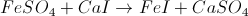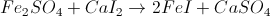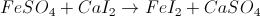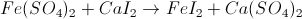Explanation:

First, we must know what ferrous sulfate is. Ferrous refers to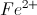, and sulfate has the formula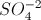. When we combine the two together we get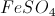.

Calcium is a divatent cation and iodide is a monovalent anion, so their salt is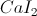. The ion exchange reaction is then:### Example Question #10 : Chemical Reactions

Select the net ionic equation from this molecular reaction: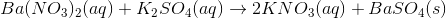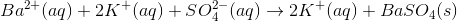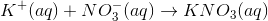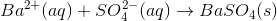None of the other choices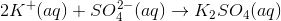Explanation:

The net ionic equation is derived by removing all spectator ions from the total ionic equation (in which all ions are listed). To put it another way, the net ionic equation involves only the ions that participate in a reaction which, in this case, is the precipitation of barium sulfate.

Begin by writing all aqueous compounds in their dissociated (ionic) forms.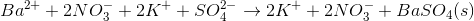Cancel out any ions that appear in equal quantities on both sides of the equation. In this case, we can cancel the nitrate and potassium ions.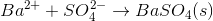This is our net ionic equation.

← Previous 1